# Understand Machine Learning with One Article

Go to the source link to view the article. You can view the picture content, updated content and better typesetting reading experience. If the link is broken, please click the button below to view the snapshot at that time.

# 1. What is Machine Learning?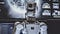Photo by Arseny Togulev on Unsplash

Broadly speaking, Machine Learning refers to the set of algorithms that learn complex patterns in a high-dimensional space. Let’s clarify this statement:

• ML models learn patterns without receiving any specific direction, as researchers impose little structure on the data. Instead, algorithms derive that structure from the data.
• We say it learns complex patterns, as the structure identified by the algorithm may not be representable as a finite set of equations.
• The term high-dimensional space refers to looking for solutions while processing a large number of variables, and the interactions between them.

For example, we could train ML algorithms to recognize human faces by showing examples and letting the model infer the patterns and structure of the face. We do not define a face, hence the algorithm learns without our directions.

There are three types of learning involved in ML:

• Supervised Learning: Learning method that consists of manually telling the model what labels the user wants to predict for the training dataset. The starting point is a set of features and resulting labels that the programmer intends the algorithm to respect. For e.g.: Regression, Classification.
• Unsupervised Learning: Learning method that consists of letting the model determine the labels and group elements of the dataset based on the more distinct features each element has. For e.g.: Clustering, Representation.
• Reinforcement Learning: Learning method in which an agent learns from the environment by interacting with it and receiving rewards for performing actions. Similarly to Supervised Learning, it receives a sort of guideline, but it’s not an input from the programmer.

## Why use it?

Machine Learning is changing every aspect of our lives. Nowadays, algorithms accomplish tasks that until recently only expert humans could perform, it’s gradually being achievable without such a level of expertise. On the other hand, traditional data analytics techniques remain static, although super useful in cases in which data structure is defined, such as this one:

## Airbnb Rental-Analysis of New York using Python

### Discover the most convenient rentals to stay implementing Data Analytics with nice visualizations.

#### towardsdatascience.com

There’s limited use for traditional data analytics in cases of fast-changing unstructured data inputs which are the most suitable for the application of techniques as Supervised and Unsupervised Learning. This is when automated processes with capacity to analyze dozens of inputs and variables emerge as precious tools.

In addition to that, the resolution process that ML techniques implement differs greatly to traditional statistics and data analytics, as they focus on receiving the input of a determined goal from the user and learning which factors are important in achieving that goal, instead of being the user who sets the factors that will determine the outcome of the target variable.

Not only it allows the algorithm to make predictions, but also compare against its predictions and adjust the accuracy of the outcome.

# 2. Popular misconceptions

Is ML the Holy Grail or is it useless?

There’s an incredible amount of hype and counter hype surrounding ML. The first one creates expectations that may not be fulfilled for the foreseeable future. On the contrary, counterhype attempts to convince audiences that there’s nothing special about ML and that classical statistics produce the same result as ML practitioners and enthusiasts claim. Both extremes prevent users and enthusiasts from recognizing the real and differentiated value that it delivers today, as it helps to overcome many of the caveats of classical techniques.

ML is a Black Box

This is the most widespread myth. Clearly it’s not fundamented as Marcos Lopez De Pradoarguments in his book, “Machine Learning for Asset Managers”, who points out that ML techniques are compatible with the scientific method as they’re been applied in every laboratory in the world to some extent, for practices such as drug development, genome research and high-energy physics. Whether someone applies it at a black box or not it’s a personal choice and surely if theory is behind the models being interpreted at first, better usage-applications will be designed.

# 3. What’s the difference between ML and Econometrics?

Econometrics objective is to infer parameters of a statistical model in order to be able to interpret variables and make conclusions based on such parameters, as they have a meaning per se. For e.g., Linear Regression coefficients indicate whether there is a positive or negative correlation between each independent variable the dependent variable. Econometrics are neither focused on predicting values of variables with a model, nor on trying to make models compete to enhance predictions in this sense.

The other side of the coin are ML analysts whose analysis is purely designed to optimize the process of prediction-making, with independence of parameter’s interpretation or model explanation, which as mentioned above is sometimes impossible to make. Although their different objectives, both disciplines are complementary and have valuable tools to be applied reciprocally.

# 4. Must-read Machine Learning Books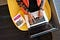Photo by Christina Morillo from Pexels

There are two types of Machine Learning potential book-readers: Practitioners and Academics. For each one of them, there’s a selection of literature to tackle, considering that the first group will essentially be looking for more applied techniques rather than focusing on the math behind the model. On the other hand, Academics might probably be looking for strict and robust empirical demonstrations behind the algorithms they will utilize.

Here is a brief recommendation for each group:

Practitioners:

# 5. Machine Learning in Finance

Financial applications pose a whole different challenge for statistics and ML because economic systems exhibit a degree of complexity that is beyond the grasp of classical statistical tools. As a consequence, ML will be increasingly playing an important role in the field, and it’s unlikely that the trend will change, as large data sets, greater computational power and more efficient algorithms are delivered to students, analysts and the community as a whole.

The main differences for applications in finance to highlight are:

• Stationarity: In finance, we work with non-stationary data, such as asset prices and other time series data sets, whereas in other scientific fields data tends to be stationary, which means that information is conditional to time periods. In addition, financial data tend to be correlated at least partially, adding more complexity to the analysis.
• Signal-to-Noise ratio: Basically, this aspect refers to how much we can predict future data, based on current input. In finance, today’s data tends to have low predictive power about future outcomes, which is not the case in general sciences such as drug development or medical applications. In these fields, experimental procedures are performed under consistent and invariable conditions in order to eliminate the uncertainty that volatility brings to the equation, such as the uncountable sources of information with which the market has to daily deal.
This issue doesn’t mean that ML cannot be applied to finance, but that it has to be applied differently.
• Interpretability of results: Due to financial regulations and compliance obligations of institutions, the argumentation of ML models application in financial strategies is a challenge for PM’s and asset managers, compared to the level of scrutiny that other scientific fields are exposed to.

In the last part, I’ll be performing a Python application of two sample models, Support Vector Machines and Linear Regression, in order to compare both of them and show in practice how a ML script is implemented. In case you don’t want to get into coding, maybe you’ll find this article useful:

# 6. Support Vector Machines and Linear Regression with Python:

Let’s dive into coding!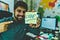Photo by hitesh choudhary from Pexels

We’ll begin to work by importing the necessary libraries: Scikit-Learn and Numpy.

Scikit-Learn is an open source machine learning library that supports supervised and unsupervised learning. It also provides various tools for model fitting, data preprocessing and model selection and evaluation, among others. In this case, we’ll be utilizing :

• Model_selection class: Includes tools for splitting dataset in train and test samples, hyper parameter optimizers and model validations.
• Linear_model class: Utilized to implement a variety of linear models such as Logistic and Linear Regressions.
• svm class: Utilized to implement supervised learning methods used for classification, regression and outliers detection.

On the other hand, Numpy is a library that provides support for large, multi-dimensional arrays and matrices, along with a large collection of high-level mathematical functions to operate on these arrays. It’s part of the foundation of the Machine Learning stack.

`# Importsimport numpy as npimport sklearn.model_selection as sk_msimport sklearn.linear_model as sk_lmimport sklearn.svm as sk_sv`

After that, we proceed with the creation of the Numpy arrays with which we’ll be working on. Consider that these will function as “synthetic” datasets, as this process could be also performed with a .csv file or a database.

First, I’ll generate a random sample of 1000 rows and 5 features that will serve as input for the model. In addition to that, I include a sample function with a predetermined array on purpose. The process that this function does consists of calculating the product of each element of the input array by every element in the X array.

`# Setting seed to lock random selectionnp.random.seed(1234)# Generate random uniform-distributed sample X = np.random.uniform(size=(1000,5))y = 2. + X @ np.array([1., 3., -2., 7., 5.]) + np.random.normal(size=1000)`

To clarify this previous code, refer to the following notebook to see the output of the created variables:

These are the shapes (length and dimension) of each array:

After we’ve created the sample datasets, we have to proceed with the split in train and test subsets that I explained in this article. This task can be performed with the already-introduced class of the scikit-learn package, called model_selection, train_test_split method: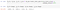In the following step, I’ll be implementing both models, the Linear Regression and Support Vector Regression. As a recap of what each model is about:

• Linear Regression: It’s a statistical model which assumes that a response variable (y) is a linear combination of weights, also known as parameters, multiplied by a set of predictor variables (x). The model includes an error to account for random sampling noise. With the term response variable, I mean that the behavior shown by the output value is dependent on another set of factors, known as predictors.
In the equation below you’ll see included β’s which are the weights, x’s which are the values of the predictor variables, and ε which is an error term representing random sampling noise or the effect of variables not included in the model.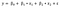• Support Vector Machines: This is also a linear model for classification and regression problems which basically consists of an algorithm that takes data as an input and outputs a line that separates observations into two classes.

In the following Gist, you’ll find the script to program both introduced models in which we can see the resulting mean of each one of them. In order to compare the models, I ran a Cross-Validation process, which is method for splitting our data into a defined number subsets, called folds (five in this case), to avoid overfitting our model:

Finally, we get to the point of evaluating the model over the testing sample and getting the output of the coefficients and the intercept: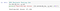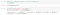# Conclusions

My aim with this article was to transmit in an easy way some of the fundamentals of Machine Learning, with a minor focus on Finance as it’s a subject that I’m personally interested in. My belief is that investors will be progressively introduced to algorithmic trading and to intelligent asset-management practices that involve AI and Machine Learning processes, so in a way this article tries to induce more people to the movement in order to be part of the change.

I hope I have achieved my goal and that it’s been useful for you! If you liked the information included in this article don’t hesitate to contact me to share your thoughts. It motivates me to keep on sharing!

# References

Thanks for taking the time to read my article! If you have any questions or ideas to share, please feel free to contact me to my email, or you can find me in the following social networks for more related content: